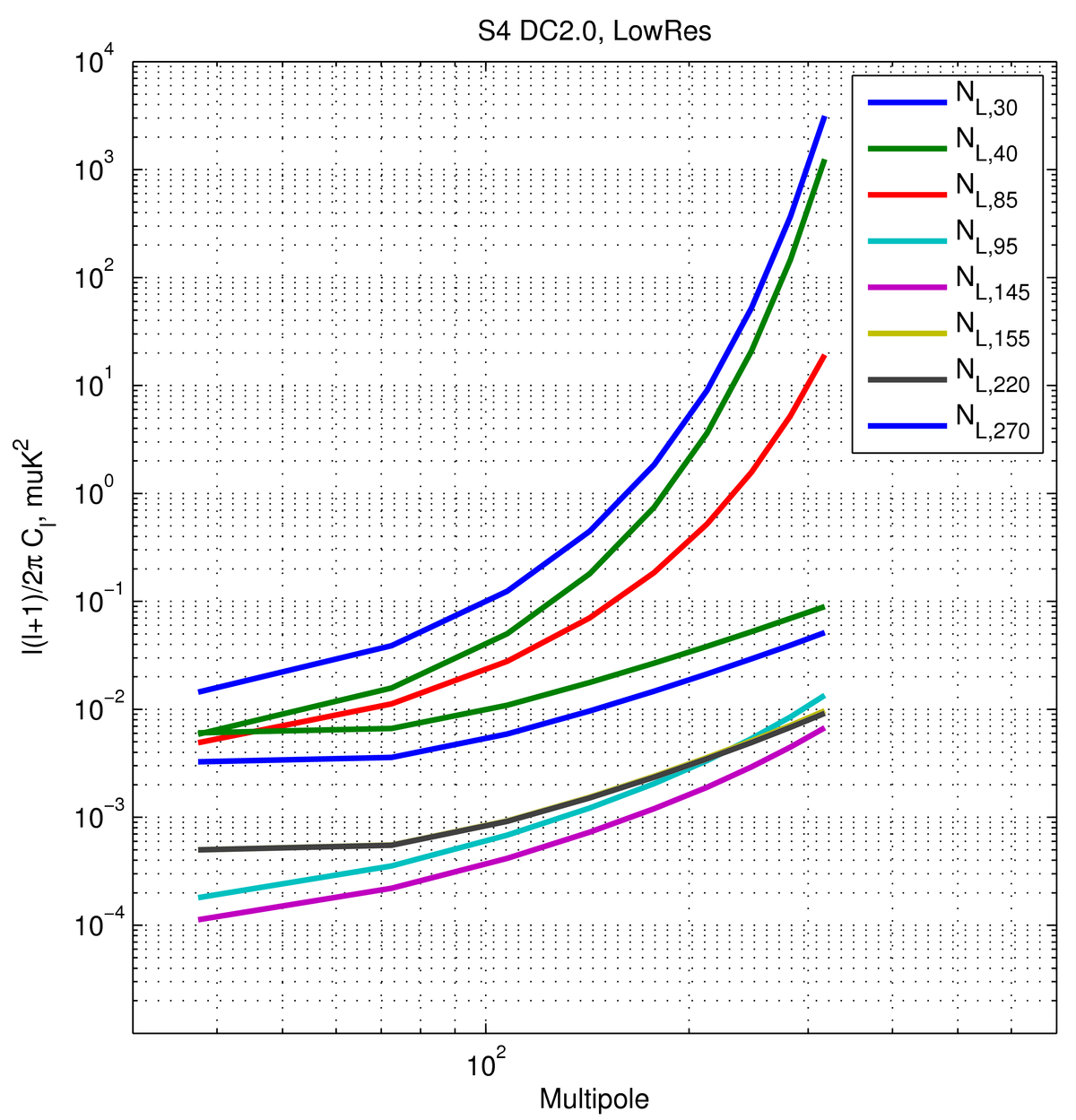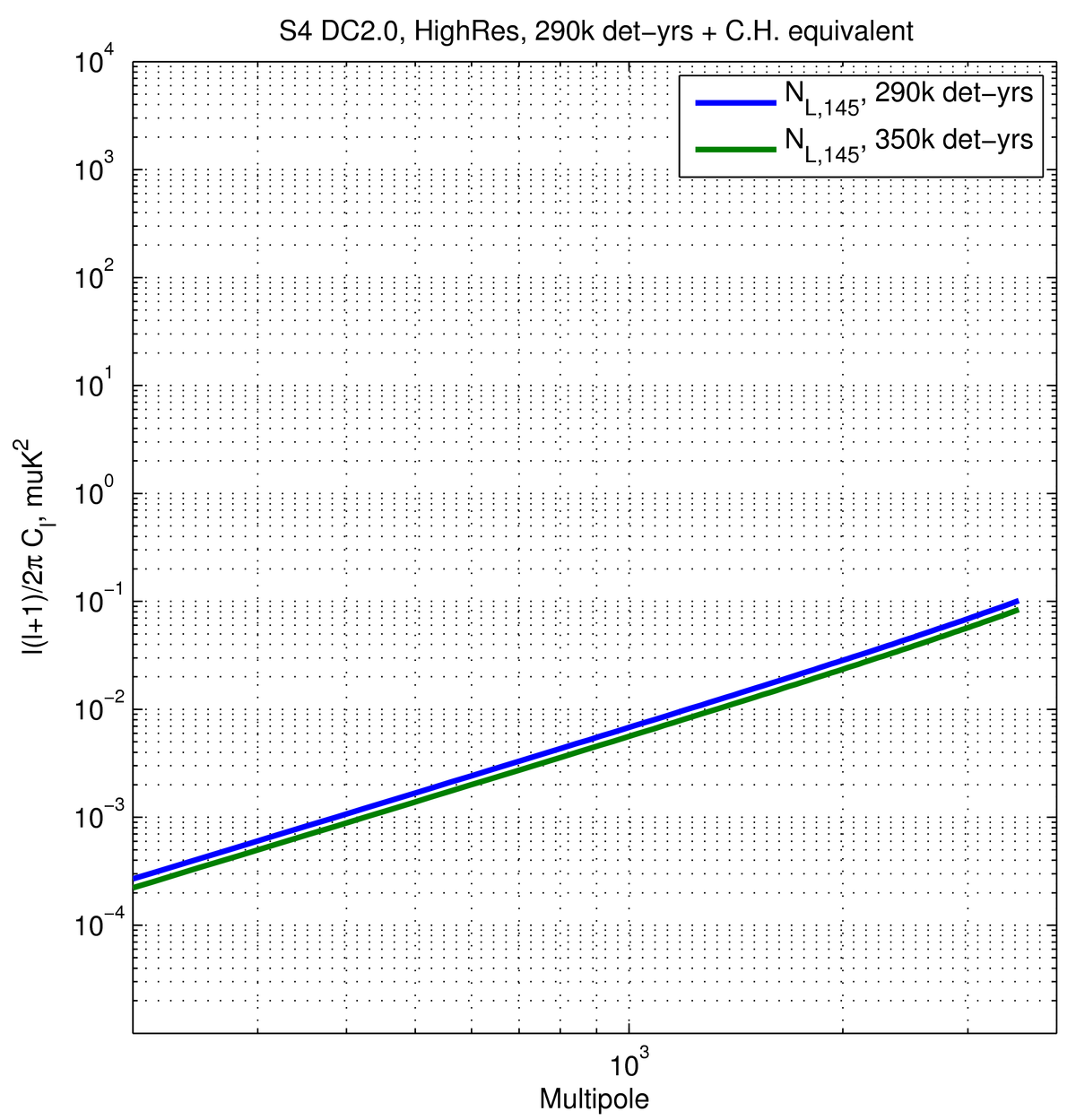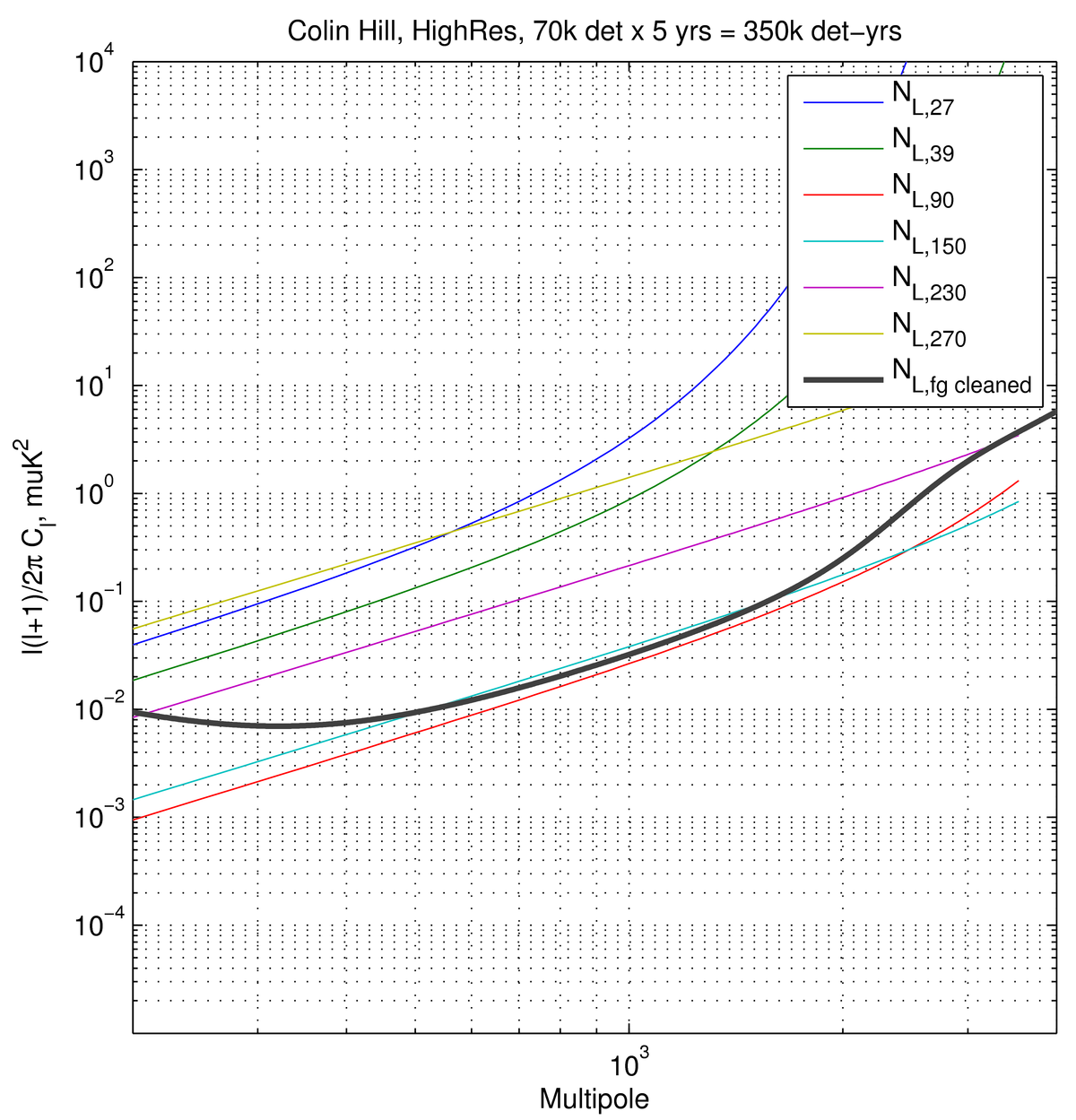High-Res studies for CMB-S4 (preliminary draft)

2017-08-04  Victor Buza

Check out Updated version HERE

Figure 1:

Left: $$N_{l}$$'s for the Degree Scale effort that went into Data Challenges 2.0 and 3.0, amounting to ~575k actual det-yrs on $$f_{sky}=0.03$$, as recorded here

Middle: $$N_{l}$$'s for the Arminute Scale effort that went into the optimization for DC2.0 and DC3.0 (though the data challenges ultimately split this effort into three bands), amounting to ~290k actual det-yrs (at 145 GHz) on $$f_{sky}=0.03$$, as recorded here. The high-res details are recorded under Table 1 in this posting.
In short: 1 arcmin beam, $$l_{min}=300$$ and $$l_{max}=4000$$ for the Phi-reconstruction, $$l_{min}=30$$ for the complete E-mode map.

In addition, I also add a 350k det-yr case for comparison with the plot to the right.

Right: Input white $$N_{l}$$'s and output foreground cleaned $$N_{l}$$ (assuming a fg cleaning method described in the posting linked to the right) for 350k det-yrs on $$f_{sky}=0.03$$, as recorded here.

At a glance conclusions: the ratio of the green $$N_l$$ curve in the middle plot and the black curve in the right plot at $$l=1000$$ is ~5, at $$l=2000$$ is ~15, and at $$l=3000$$ is ~30. Perhaps this is mostly due to the fact that C.H.'s ILC tries to completely zero out the fg contributions at high ell and therefore spends a lot of effort in trying to do so. We therefore expect that if one were to use Colin's fg cleaned $$N_l$$ to obtain a delensing fraction, it will be quite a bit lower than the one assumed in the Science Book and data-challenges.